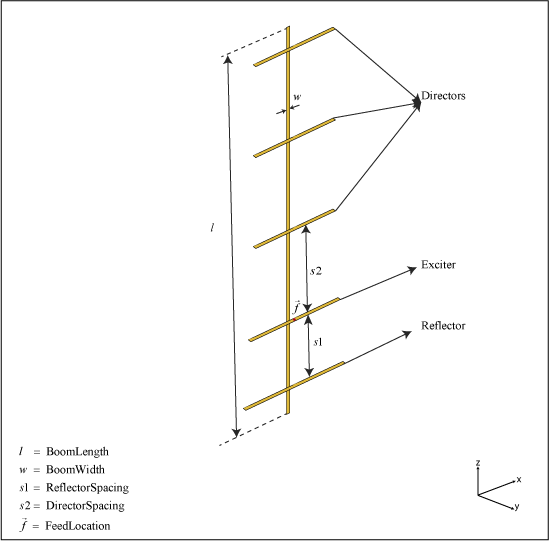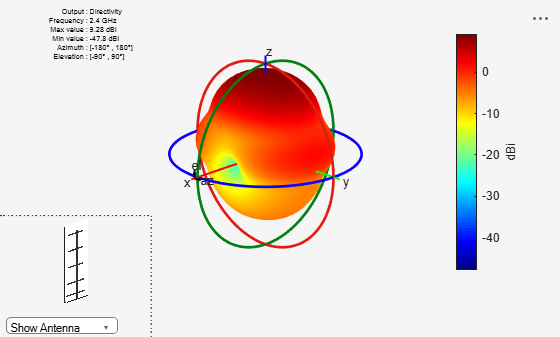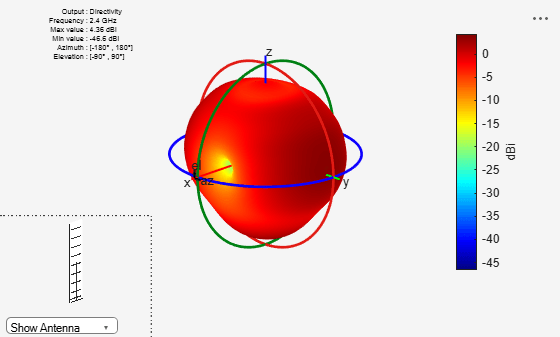Create Yagi-Uda custom array antenna

## Description

The `quadCustom` object creates a Yagi-Uda custom array along the Z-axis.## Creation

### Syntax

``ant = quadCustom``
``ant = quadCustom(Name,Value)``

### Description

example

````ant = quadCustom` creates a half-wavelength Yagi-Uda custom array antenna along the Z-axis. The default antenna is excited using a dipole and consists of three directors and one reflector. The default dimensions are chosen for an operating frequency of 2.4 GHz.```
````ant = quadCustom(Name,Value)` sets properties using one or more name-value pairs. For example, `ant = quadCustom('Exciter',dipoleFolded)` creates a Yagi-Uda custom array antenna with a folded dipole antenna as the exciter.```

### Output Arguments

expand all

Yagi-Uda custom array antenna, returned as a `quadCustom` object.

## Properties

expand all

Antenna type used as an exciter, specified as a `dipoleFolded`, `biquad`, `dipole`, or `loopCircular` antenna object. This `quadCustom` supports a single exciter.

Example: `'Exciter',dipoleFolded`

Example: `ant.Exciter = dipoleFolded`

Antenna type or antenna shape used as director elements, specified as a cell array consisting of one or more of the following antennas: `dipole`, `dipoleVee`, `biquad`, `loopRectangular`, `loopCircular`, `antenna.Polygon`, `antenna.Circle`, or `antenna.Rectangle`. You can use single or multiple antenna elements as directors.

Example: ```d = dipoleVee; ant = quadCustom('Director',{d d d d})```. Yagi-Uda custom array antenna uses V-dipole as its directors.

Example: ```d = dipoleVee; ant = quadCustom; ant.Director= {d d d d} ```. Yagi-Uda custom array antenna uses V-dipole as its directors.

Spacing between the director elements, specified as a real-valued scalar in meters or a vector with each element unit in meters. You can specify a scalar value for equal spacing between the elements and vector value for unequal spacing between the elements. If you use a vector, the first value is the distance between the exciter and the first director element.

Example: `'DirectorSpacing',[0.234 0.324]`

Example: ```ant.DirectorSpacing = [0.234 0.324]```

Data Types: `double`

Antenna type used as reflector elements, specified as a cell array. You can use single or multiple antenna elements as reflectors.

Example: ```d = dipoleVee;ant = quadCustom('Reflector',{d d d d})``` Yagi-Uda custom array antenna uses V- dipole as its reflectors.

Example: ```d = dipoleVee;ant = quadCustom;ant.Reflector={d d d d}``` Yagi-Uda custom array antenna uses V- dipole as its reflectors.

Spacing between the reflector elements, specified as a real-valued scalar in meters or a vector with each element unit in meters. You can specify a scalar value for equal spacing between the elements or a vector value for unequal spacing between the elements. If you use a vector, the first value is the distance between the exciter and the first reflector element.

Example: `'ReflectorSpacing',[0.234 0.324]`

Example: ```ant.ReflectorSpacing = [0.234 0.324]```

Data Types: `double`

Length of the boom, specified as a real-valued scalar in meters.

Example: `'BoomLength',0.234`

Example: `ant.BoomLength = 0.234`

Data Types: `double`

Width of the boom, specified as a real-valued scalar in meters.

Example: `'BoomWidth',0.00324`

Example: `ant.BoomWidth = 0.00324`

Data Types: `double`

Signed distance from center of antenna elements, specified as a three-element vector with each element unit in meters.

Example: `'BoomOffset',[0 0.0060 0.0350]`

Example: ```ant.BoomOffset = [0 0.0060 0.0350]```

Data Types: `double`

Lumped elements added to the antenna feed, specified as a lumped element object handle. You can add a load anywhere on the surface of the antenna. By default, the load is at the feed. For more information, see `lumpedElement`.

Example: `'Load',lumpedelement`. `lumpedelement` is the object handle for the load created using `lumpedElement`.

Example: ```ant.Load = lumpedElement('Impedance',75)```

Tilt angle of the antenna, specified as a scalar or vector with each element unit in degrees. For more information, see Rotate Antennas and Arrays.

Example: `'Tilt',90`

Example: `ant.Tilt = 90`

Example: `'Tilt',[90 90]`,`'TiltAxis',[0 1 0;0 1 1]` tilts the antenna at 90 degrees about the two axes, defined by vectors.

### Note

The `wireStack` antenna object only accepts the dot method to change its properties.

Data Types: `double`

Tilt axis of the antenna, specified as:

• Three-element vectors of Cartesian coordinates in meters. In this case, each vector starts at the origin and lies along the specified points on the X-, Y-, and Z-axes.

• Two points in space, each specified as three-element vectors of Cartesian coordinates. In this case, the antenna rotates around the line joining the two points in space.

• A string input describing simple rotations around one of the principal axes, 'X', 'Y', or 'Z'.

Example: `'TiltAxis',[0 1 0]`

Example: `'TiltAxis',[0 0 0;0 1 0]`

Example: `ant.TiltAxis = 'Z'`

### Note

The `wireStack` antenna object only accepts the dot method to change its properties.

## Object Functions

 `show` Display antenna or array structure; display shape as filled patch `axialRatio` Axial ratio of antenna `beamwidth` Beamwidth of antenna `charge` Charge distribution on metal or dielectric antenna or array surface `current` Current distribution on metal or dielectric antenna or array surface `design` Design prototype antenna or arrays for resonance at specified frequency `EHfields` Electric and magnetic fields of antennas; Embedded electric and magnetic fields of antenna element in arrays `impedance` Input impedance of antenna; scan impedance of array `mesh` Mesh properties of metal or dielectric antenna or array structure `meshconfig` Change mesh mode of antenna structure `pattern` Radiation pattern and phase of antenna or array; Embedded pattern of antenna element in array `patternAzimuth` Azimuth pattern of antenna or array `patternElevation` Elevation pattern of antenna or array `returnLoss` Return loss of antenna; scan return loss of array `sparameters` S-parameter object `vswr` Voltage standing wave ratio of antenna

## Examples

collapse all

Create and view a custom Yagi-Uda array antenna.

`ant = quadCustom`
```ant = quadCustom with properties: Exciter: [1x1 dipole] Director: {[1x1 dipole] [1x1 dipole] [1x1 dipole]} DirectorSpacing: 0.0423 Reflector: {[1x1 dipole]} ReflectorSpacing: 0.0308 BoomLength: 0.1800 BoomWidth: 0.0020 BoomOffset: [0 0.0050 0.0450] Tilt: 0 TiltAxis: [1 0 0] Load: [1x1 lumpedElement] ```
`show(ant)`Plot the radiation pattern of the antenna at 2.4 GHz.

`pattern(ant,2.4e9)`Create the default quadCustom, change the number of directors to seven, and view the structure.

``` ant = design(dipole,2.4e9); ant.Tilt = 90```
```ant = dipole with properties: Length: 0.0587 Width: 0.0012 FeedOffset: 0 Tilt: 90 TiltAxis: [1 0 0] Load: [1x1 lumpedElement] ```
` ant.TiltAxis = [0 1 0]`
```ant = dipole with properties: Length: 0.0587 Width: 0.0012 FeedOffset: 0 Tilt: 90 TiltAxis: [0 1 0] Load: [1x1 lumpedElement] ```
` quad_ant = quadCustom('Director',{ant,ant,ant,ant,ant,ant,ant})`
```quad_ant = quadCustom with properties: Exciter: [1x1 dipole] Director: {1x7 cell} DirectorSpacing: 0.0423 Reflector: {[1x1 dipole]} ReflectorSpacing: 0.0308 BoomLength: 0.1800 BoomWidth: 0.0020 BoomOffset: [0 0.0050 0.0450] Tilt: 0 TiltAxis: [1 0 0] Load: [1x1 lumpedElement] ```
` show(quad_ant)`Plot the radiation pattern of the antenna at the frequency 2.4 GHz.

`pattern(quad_ant,2.4e9)`Bankey, Vinay, and N.Anvesh Kumar. "Design of a Yagi-Uda Antenna with Gain and Bandwidth Enhancement for Wi-Fi and Wi-Max Applications." International Journal of Antennas. Vol.2, Number 1, 2017